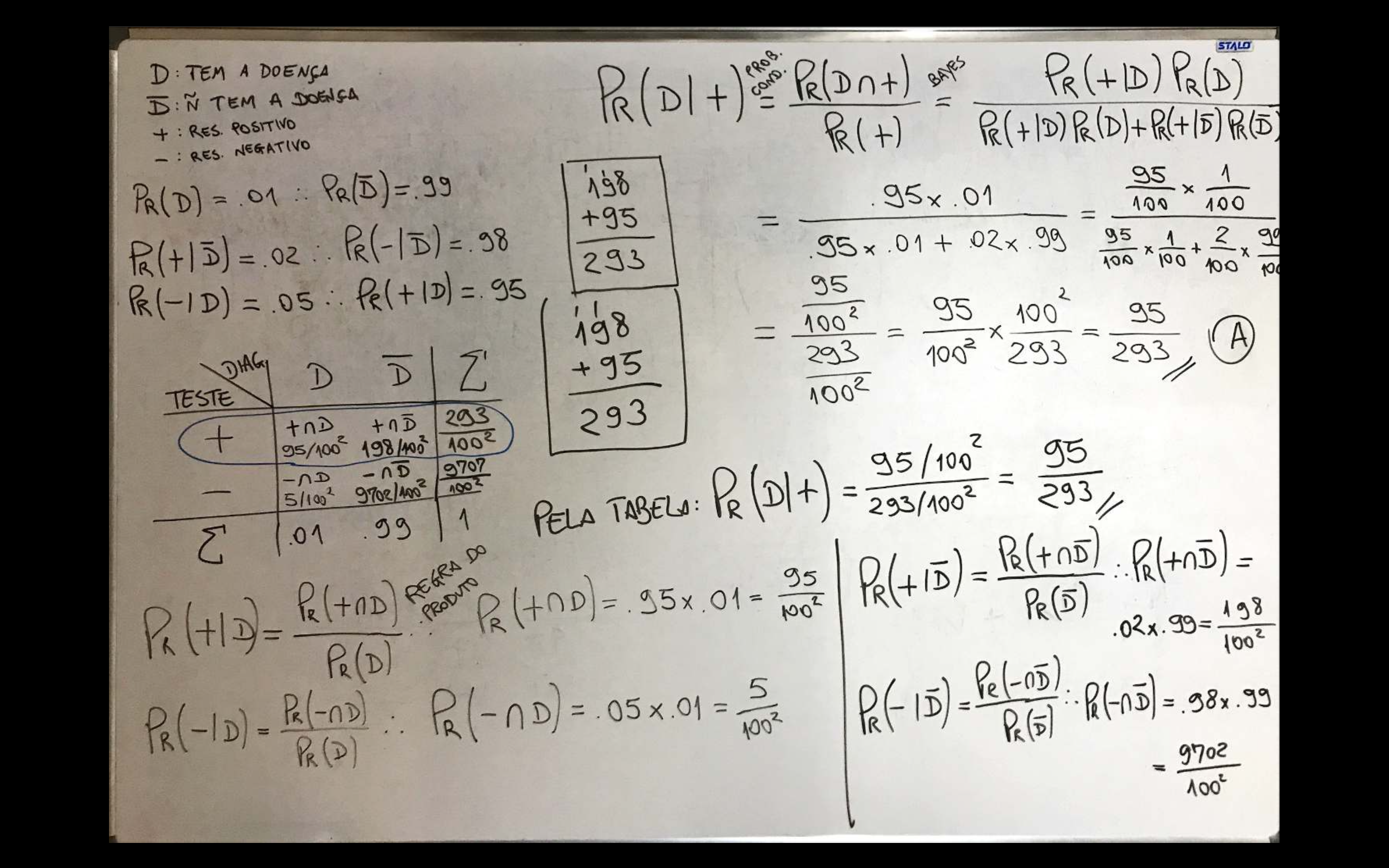## 8.3 Chapter 3

Solution. ??

1. $$\Omega_{4} = \{ 1,2,3,4 \}$$
$$\Omega_{6} = \{ 1,2,3,4,5,6 \}$$
$$\Omega_{8} = \{ 1,2,3,4,5,6,7,8 \}$$
$$\Omega_{10} = \{ 1,2,3,4,5,6,7,8,9,10 \}$$
$$\Omega_{12} = \{ 1,2,3,4,5,6,7,8,9,10,11,12 \}$$
$$\Omega_{20} = \{ 1,2,3,4,5,6,7,8,9,10,11,12,13,14,15,16,17,18,19,20 \}$$
$$\Omega_{100} = \{ 10,20,30,40,50,60,70,80,90,100 \}$$

2. $\Omega_{4,4} = \left\lbrace \begin{array}{cccccc} (1,1) & (1,2) & (1,3) & (1,4) \\ (2,1) & (2,2) & (2,3) & (2,4) \\ (3,1) & (3,2) & (3,3) & (3,4) \\ (4,1) & (4,2) & (4,3) & (4,4) \\ \end{array} \right\rbrace$

3. $\Omega_{4,6} = \left\lbrace \begin{array}{cccccc} (1,1) & (1,2) & (1,3) & (1,4) & (1,5) & (1,6) \\ (2,1) & (2,2) & (2,3) & (2,4) & (2,5) & (2,6) \\ (3,1) & (3,2) & (3,3) & (3,4) & (3,5) & (3,6) \\ (4,1) & (4,2) & (4,3) & (4,4) & (4,5) & (4,6) \\ \end{array} \right\rbrace$

Solution. 3.4
a. $$Pr(C) = \frac{1}{308,000} \approx 0.0000032468$$
b. $$Pr(H) = \frac{1}{17,600} \approx 0.0000568182$$
c. $$Pr(M) = \frac{1}{10,600} \approx 0.0000943396$$
d. $$Pr(C \cup H \cup M) = \frac{1}{308,000} + \frac{1}{17,600} + \frac{1}{10,600} = \frac{5,041}{32,648,000} \approx 0.0001544046$$
e. $$8,000,000,000 \times \frac{1}{308,000} \approx 25,974$$
$$8,000,000,000 \times \frac{1}{17,600} \approx 454,545$$
$$8,000,000,000 \times \frac{1}{10,600} \approx 754,717$$
$$8,000,000,000 \times \frac{5,041}{32,648,000} \approx 1,235,236$$

Solution. ??
a. $$\frac{1}{2} + \frac{1}{3} + \frac{1}{9}= \frac{17}{18} < 1$$
b. Since the remainder of dividing 35 by 3 is 2, there are 2 camels left over no matter how the division is done. Note that $$18+12+4=34$$.

Solution. 3.6

• P4 (Complement)
By (3.22) $Pr(\Omega) = 1$ You can write $$\Omega = A \cup A^c$$, thus $Pr(A \cup A^c) = 1$ As $$A \cap A^c = \emptyset$$, by (3.23)
$Pr(A \cup A^c) = Pr(A) + Pr(A^c)$ Thus, $Pr(A) + Pr(A^c) = 1 \therefore Pr(A) = 1 - Pr(A^c)$ $$\bigtriangleup$$

• P5

• P6
$$A \cup B = A \cup (B-A)$$ e $$A \cap (B-A) = \emptyset$$. Thus, by (3.23)
$\begin{equation} Pr(A \cup B) = Pr(A) + Pr(B-A) \tag{1} \end{equation}$ As $$A \subset B$$, $$B = (B-A) \cup (A \cap B)$$ and $$(B-A) \cap (A \cap B) = \emptyset$$. Thus, again by (3.23)
$\begin{equation} Pr(B) = Pr(B-A) + Pr(A \cap B) \tag{2} \end{equation}$ Combining (1) and (2), $Pr(A \cup B) = Pr(A) + Pr(B) - Pr(A \cap B).$ $$\bigtriangleup$$

• P7

• P8

Solution. 3.10Solution. 3.12

1. $$R_D = \{ -5,-4,-3,-2,-1,0,1,2,3,4,5 \}$$

2. $$R_P = \{ 1,2,3,4,5,6,8,9,10,12,15,16,18,20,24,25,30,36 \}$$

3. $$R_Q = \left\{ \frac{1}{6},\frac{1}{5},\frac{1}{4},\frac{1}{3},\frac{2}{5},\frac{1}{2},\frac{3}{5},\frac{2}{3},\frac{3}{4},\frac{4}{5},\frac{5}{6},1,\frac{6}{5},\frac{5}{4},\frac{4}{3},\frac{3}{2},\frac{5}{3},2,\frac{5}{2},3,4,5,6 \right\}$$

Solution. 3.13

1. $$R_Y = \{ -5,-4,-3,-2,-1,0,1,2,3,4,5 \}$$
$$Pr(Y=-5) = Pr(Y=5) = \frac{1}{36}$$
$$Pr(Y=-4) = Pr(Y=4) = \frac{2}{36}$$
$$Pr(Y=-3) = Pr(Y=3) = \frac{3}{36}$$
$$Pr(Y=-2) = Pr(Y=2) = \frac{4}{36}$$
$$Pr(Y=-1) = Pr(Y=1) = \frac{5}{36}$$
$$Pr(Y=0) = \frac{6}{36}$$
De forma geral, $$Pr(Y=y) = \frac{6-|y|}{36}$$.

Solution. 3.14

Solution. 3.17

1. It is know that $$\sum_{x=0}^{\infty} \frac{\lambda^x}{x!} = e^\lambda$$. Thus,

\begin{align*} \sum_{x=0}^{\infty} \left[ p \frac{e^{-\lambda_1} \lambda_{1}^{x}}{x!} + (1-p) \frac{e^{-\lambda_2} \lambda_{2}^{x}}{x!} \right] =& p e^{-\lambda_1} \sum_{x=0}^{\infty} \frac{\lambda_{1}^{x}}{x!} + e^{-\lambda_2} \sum_{x=0}^{\infty} \frac{\lambda_{2}^{x}}{x!} - p e^{-\lambda_2} \sum_{x=0}^{\infty} \frac{\lambda_{2}^{x}}{x!} \\ =& p e^{-\lambda_1} e^{\lambda_1} + e^{-\lambda_2} e^{\lambda_{2}} - p e^{-\lambda_2} e^{\lambda_{2}} \\ =& p + 1 - p \\ =& 1 \end{align*} $$\bigtriangleup$$

Solution. 3.24

pnorm(-1.96) # Pr(Z ≤ -1.96), green area
##  0.0249979
pnorm(1)-pnorm(-1) # Pr(-1 ≤ Z ≤ 1), blue area
##  0.6826895
1-pnorm(1.64) # Pr(Z > 1.64), red area
##  0.05050258
qnorm(0.025) # q such that Pr(Z ≤ q) = 0.025, green area
##  -1.959964
qnorm((1-0.6826894921)/2)  # q such that Pr(-q ≤ Z ≤ q) = 0.6826894921, blue area
##  -1
qnorm(0.05, lower.tail = F) # q such that Pr(Z > q) = 0.05, red area
##  1.644854

Solution. 3.26

1. $$F(X) = 1-e^{-2x}$$

2. Pr(X<1) = F(1) = 1-e^{-2 } = 1-e^{-2}

3. Pr(X>2) = 1-F(2) = 1-(1-e^{-2 }) = e^{-4}

curve(dexp(x), 0, 4)